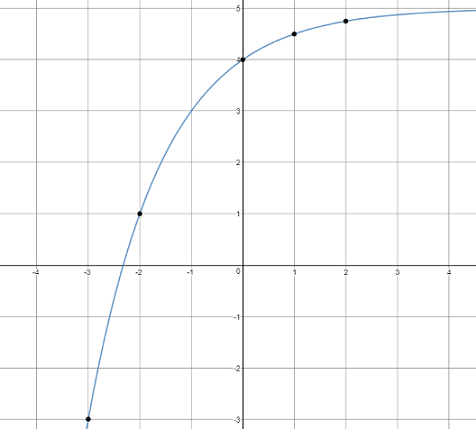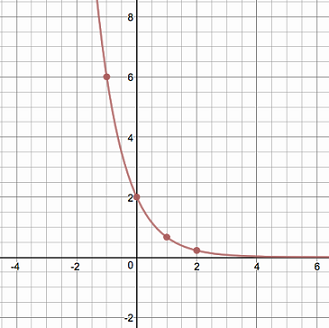# Exponents and Exponential Functions

## Objective

Write, graph, and evaluate exponential decay functions.

## Common Core Standards

### Core Standards

?

• F.IF.C.8.B — Use the properties of exponents to interpret expressions for exponential functions. For example, identify percent rate of change in functions such as y = (1.02)t, y = (0.97)t, y = (1.01 12t, y = (1.2)t/10, and classify them as representing exponential growth or decay.

• F.LE.A.1.C — Recognize situations in which a quantity grows or decays by a constant percent rate per unit interval relative to another.

?

• 8.F.B.4

## Criteria for Success

?

1. Understand that an exponential decay function has a decreasing rate of change, represented by a value between $0$ and $1$, compared to an exponential growth function that has an increasing rate of change.
2. Identify exponential decay functions in equations, tables, and graphs.
3. Analyze an exponential decay function in equation form to identify features in the graph of the function including the $y$-intercept, domain, range, rate of change, etc.
4. Analyze the graph of an exponential decay function to write an equation for the function.

## Anchor Problems

?

### Problem 1

Compare and contrast functions ${{f(x)}}$ and ${{g(x)}}$ by answering the questions below.

${{f(x)}}=\left({1\over2}\right)^x$

${{g(x)}}=(2)^x$

a.   What happens to the values of ${{f(x)}}$ and ${{g(x)}}$ as $x$ increases?

b.   What are the domains of ${{f(x)}}$ and ${{g(x)}}$?

c.   What are the ranges of ${{f(x)}}$ and ${{g(x)}}$?

d.   Create a table of values for each function and use them to sketch a graph of each function.

### Problem 2

Consider the exponential graph below.1. Create a table of values for the function.
2. Does the function represent exponential growth or exponential decay? Explain your reasoning.
3. What transformations were applied to the graph of this function? Explain your reasoning.
4. Determine the equation for this function.

### Problem 3

Sketch a graph of each function shown below.

${f(x)=2(3)^{-x}}$

${g(x)=\left(1\over2\right)^x-3}$

## Problem Set

?

The following resources include problems and activities aligned to the objective of the lesson that can be used to create your own problem set.

• Include problems where students graph exponential decay functions, including those with transformations

Graph the exponential function ${f(x)=-\left({1\over2}\right)^x}$.